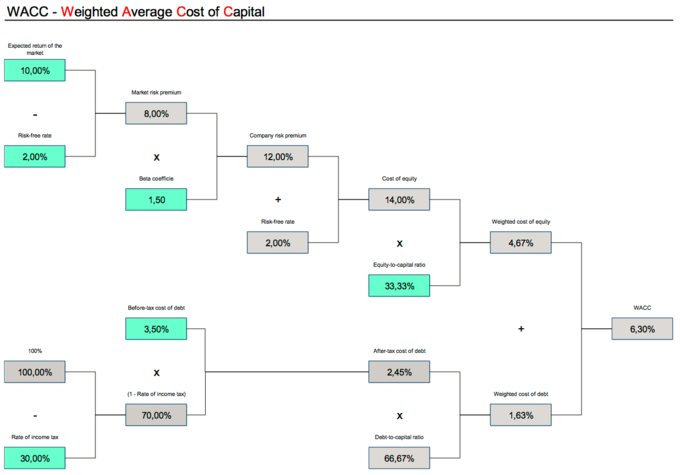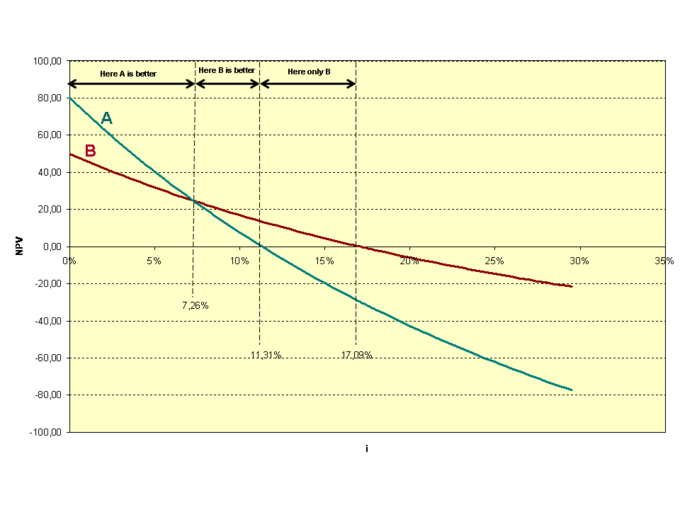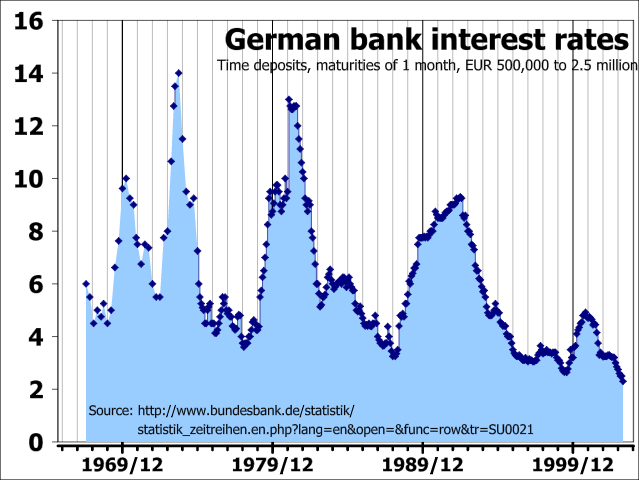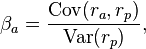## Weighted Average Cost of Capital

The weighted average cost of capital (WACC) is a calculation that reflects how much an organization pays in interest when acquiring financing options.

### Learning Objectives

Derive the weighted average cost of capital

### Key Takeaways

#### Key Points

• The weighted average cost of capital (WACC) is a calculation that allows firms to understand the overall costs of acquiring financing.
• Capital inputs generally come in the form of debt and equity. Debt is usually quite simple to calculate as it is set in the terms of bonds and loans explicitly.
• Equity is a bit more complex, as it is subjected to market (systematic) risk. The capital asset pricing model is a useful tool in estimating the cost of equity.
• Applying the WACC to the estimated rate of return for new projects and ventures is a simple way to determine if a project is sufficiently profitable to offset the cost (risk) of financing.

#### Key Terms

• weighted average cost of capital: A calculation of the overall cost of capital used by an enterprise, made by totaling the cost of each source of capital used multiplied by its proportional share of the total capital used.

### Weighted Average Cost of Capital Defined

Organizations have a few options available when it comes to finding funding for their operations. From debt options such as taking out loans or offering long-term corporate bonds to equity such as preferred and common stock, larger organizations tend to find a balance between these options that is optimized for the best possible weighted average cost of capital (WACC) to operate at the scale that creates the best revenue opportunity.

In short, the WACC is a measure of what all of these capital inputs will cost the organization in terms of an average interest rate.

### Why Calculate WACC?

WACC is a useful calculation, as it shows management what the cost of borrowing capital is overall. This overall cost of capital can then be a minimum required return on any new operation. For example, if it will cost 8% in capital costs to fund a project that creates 10% in profit, the organization can confidently borrow capital to fund this project. If the project would only turn 8% profit, the firm would have a difficult decision. If the project would turn 6% profit, it is quite easy to strategically argue against the new project.

### How to Calculate WACC

Calculating the cost of capital is actually quite a simple equation. Most firms are only receiving from either debt or equity (though there can be quite few inputs to each of these subheadings). What this means is that the overall percentage of the business that is funded by debt (MVd/MVd + MVe) must be multiplied by the cost of debt (Rd),  while similarly the percent funding of equity (MVe/MVd + MVe) must be multiplied by the cost of equity (Re) and added together. This can also be applied to the corporate tax rate in a given country of operation. This is written out as follows:

${\text{WACC}}={\frac {\text{MV}_{\text{e}}}{\text{MV}_{\text{d}}+\text{MV}_{\text{e}}}}\cdot \text{R}_{\text{e}}+{\frac {\text{MV}_{\text{d}}}{\text{MV}_{\text{d}}+\text{MV}_{\text{e}}}}\cdot \text{R}_{\text{d}}\cdot (1-\text{t})$

The cost of debt is usually fixed, based on the terms of a given bond or loan contract. As a result, the cost of debt is usually both certain and predictable. The cost of equity is a little bit more complex, as it is speculative and often determined (to some degree) by investor behavior. The capital asset pricing model (CAPM) is a traditional approach to determining the cost of equity:

$\text{E}(\text{R}_{\text{i}})=\text{R}_{\text{f}}+\beta _{\text{im}}(\text{E}(\text{R}_{\text{m}})-\text{R}_{\text{f}}).\,$

Each of the variables here are defined as:

• Es – expected return
• Rf – expected risk-free return
• βs – sensitivity to market risk
• Rm -historical return
• (Rm – Rf) – risk premium of market assets over risk free assets.

By calculating the estimated cost of equity, and applying that to the WACC equation with the cost of debt and capital structure, organizations can determine the cost of capital (and thus the required return on projects/assets).Weighted Average Cost of Capital Tree: This diagram is an excellent illustration of how various forms of debt and equity consolidate into broader calculation of debt and equity overall, and how those can combine as a total weighted average cost of capital.

## The Weightings

The weightings used in the WACC are ratios of the market values of various forms of debt and equity used in a company’s financing.

### Learning Objectives

Define how a company’s weighted average cost of capital is weighted

### Key Takeaways

#### Key Points

• The WACC must take into account the weight of each component of a company’s capital structure.
• The calculation of the WACC usually uses the market values of the various components rather than their book values.
• Market value is the price at which an asset would trade in a competitive auction setting.
• Book value refers to the value of an asset according to the account balance present on the balance sheet of a company.
• If the value of a company’s debt exceeds the value of its equity, the cost of its debt will have more “weight” in calculating its total cost of capital than the cost of equity. If the value of the company’s equity exceeds its debt, the cost of its equity will have more weight.

#### Key Terms

• Synergy: Benefits resulting from combining two different groups, people, objects, or processes.

### The Weightings

To calculate the weighted average cost of capital (WACC) we must take into account the weight of each component of a company’s capital structure. To review, the equation for WACC is:

$\text{WACC}\quad =\quad \frac { \text{MV}_\text{e} }{ \text{MV}_\text{d}+\text{MV}_\text{e} } \cdot\text{R}_\text{e}+\frac { \text{MV}_\text{d} }{ \text{MV}_\text{d}+\text{MV}_\text{e} } \cdot\text{R}_\text{d}\cdot(1-\text{t})$

MVe stands for the market value of equity; MVd stands for the Market Value of Debt; Re stands for cost of equity; Rd stands for cost of debt; and t is the company’s tax rate. If the person analyzing a company chooses or if the market value of a company’s debt and equity is not available, the book value can be used.

The “weighting” varies based on how the company finances its activities. If the value of a company’s debt exceeds the value of its equity, the cost of its debt will have more “weight” in calculating its total cost of capital than the cost of equity. If the value of the company’s equity exceeds its debt, the cost of its equity will have more weight.

### Market Value vs. Book Value

Since we are measuring expected cost of new capital, the calculation of weighted average cost of capital usually uses the market values of the various components rather than their book values. These may differ significantly.

Market value is the price at which an asset would trade in a competitive auction setting. It is the true underlying value of an asset according to theoretical standards. It is a distinct concept from market price, which is the price at which one can transact. For market price to equal market value, the market must be efficient and rational. Market value also requires the element of “special value” to be disregarded. Special value refers to a synergy that may exist between two parties that makes the fair price of a transaction higher.

Book value refers to the value of an asset according to the account balance present on the balance sheet of a company. The balance sheet is a summary of the financial balances of a company and is often described as a “snapshot of a company’s financial condition. ”

An asset’s initial book value is its actual cash value or its acquisition cost. Cash assets are recorded or “booked” at actual cash value. Assets such as buildings, land, and equipment are valued based on their acquisition cost, which includes the actual cash cost of the asset, plus certain costs tied to the purchase of the asset, such as broker fees.Domestic Balance Sheet: If the person analyzing a company chooses or if the market value of a company’s debt and equity is not available, the book value can be used. The book value of debt and equity can be found on the company’s balance sheet.

## Factors Controlled by the Firm

Decisions about capital structure (ratio of debt and equity) alongside projecting rates of return can give firms some internal control over capital costs.

### Learning Objectives

Recognize the strategic considerations of capital structure by understanding weighted average cost of capital and the internal rate of return

### Key Takeaways

#### Key Points

• By understanding the weighted average cost of capital (WACC) and potential internal rates of return (IRR) of various projects, firms can have some strategic control of capital costs and investment returns.
• Each funding source will come with a different cost of capital. Averaging these funding sources into the WACC is a central tool for financial management.
• Once the costs of capital are optimized, a business can apply this cost of capital to various asset and project assessments. The IRR must exceed the WACC.

#### Key Terms

• capital structure: The way that a corporation finances its assets through some combination of equity, debt, and hybrid securities.

When pursuing financing, organizations encounter a variety of factors that impact the weighted average cost of capital. Some of these factors are within the firm’s strategic control, while others are external forces outside the firm’s control. As a financial professional or upper level strategist, understanding what capital structure options are available to a firm plays a critical role in financial management.

### Capital Structure

Capital structure refers to the way in which an organization finances operations. This is generally illustrated via a balance sheet, where the overall assets are offset by the capital structure of liabilities and equity. It is through the decisions to acquire various forms of debt and equity that an organization can derive a weighted average cost of capital (WACC) that is sustainable within the context of organizational profitability. If the cost of capital is higher than the returns from those investments, the organization lacks the profitability required to justify itself. WACC is calculated as follows:

${\text{WACC}}={\frac {\text{MV}_{\text{e}}}{\text{MV}_{\text{d}}+\text{MV}_{\text{e}}}}\cdot \text{R}_{\text{e}}+{\frac {\text{MV}_{\text{d}}}{\text{MV}_{\text{d}}+\text{MV}_{\text{e}}}}\cdot \text{R}_{\text{d}}\cdot (1-\text{t})$

In the above equation, the first segment is measuring the cost of equity coupled with the percentage of the capital structure that is funded by equity. The second segment is making the same calculation, but this time with the cost of debt and the relative percentage of capital structure which is funded via this source. The final segment (1 – t) is the application of a corporate tax rate (depending on the country of operation.

Understanding the cost of each input of the capital structure, firms can control (to some degree) how they fund their operations and acquisitions of assets.

### Internal Rate of Return

Another important decision made by financial professionals in relation to the cost of capital revolves around the required rates of return of various projects. Investing capital into an operation always incurs the opportunity cost of investing in something else. As a result, organizations can control their operations by measuring and projecting the rate of return on each project, and investing strategically in the most profitable projects.

An internal rate of return (IRR) calculation can be useful when doing this. The IRR is going to look at the net present value (NPV) of a given project, and calculate it for a break even point (i.e. set the equation to zero, when taking cost into account). By doing so, the organization can identify the anticipated rate of return on the project. take a look at the equation below:

$\mathrm {\text{NPV}} =\sum _{\text{n}=0}^{\text{N}}{\frac {\text{C}_{\text{n}}}{(1+\text{r})^{\text{n}}}}=0$

In this equation, n is the number of periods in a project (i.e. number of anticipated cash flows over the life of the project), C is the cash flow amount (which can vary for each period, hence the summation), r is the rate of return being solved for, and N is total number of periods (n). When solving for zero, the organization will identify what the rate of return is for each project. Deciding upon projects that provide returns greater than the WACC derived from the capital structure will set the organization down a profitable path (assuming forecasts are reliable!).Exclusive_investments.png: When weighed different projects over time, the IRR calculation can be useful in determining which investment of capital is best over the lifetime of each project. When taking the WACC into account (derived from capital structure decisions), the organization can measure operational options against the required rate of return.

## Factors External to the Firm

The weighted average cost of capital is vulnerable to market risks, interest rate changes, inflation, economic factors, and tax rates.

### Learning Objectives

Recognize the various external factors that may impact the weighted average cost of capital

### Key Takeaways

#### Key Points

• While organizations have some control over capital structure decisions, there are many factors at play in the external environment that are outside the control of the firm.
• Interest rates, inflation, and other economic forces can play a substantial role in the overall cost of capital.
• Fluctuations in the marketplace (i.e. market risk, or systematic risk) can be both unpredictable and influential on overall risk and return for investors, impacting capital costs.
• Corporate and investor tax legislation impacts the cost of capital, as both organizations and investors must factor tax into their investment decisions.

#### Key Terms

• systematic risk: Risk that is intrinsic to the marketplace.

When organizations begin weighing the weighted average cost of capital (WACC) and determining overall capital structure, there are some factors that are within the control of the organization, and some external factors that are not. When it comes to projecting the cost of capital, it’s useful to assess some of the external factors that may influence the overall cost.

### Interest Rates

Interest rates fluctuate for a wide variety of reasons, and are a primary tool of monetary policy. Governments and central banks often make alterations to interest rates as a result of investment, unemployment, inflation, and other broader economic factors. Altering interest rates can impact the spending, borrowing, and investing habits within an economy, and therefore tend to fluctuate for economic purposes.German Bank Interest Rates: This chart illustrates changes in interest rates in Germany over time.

### Inflation

Related to interest rates (and a direct impact upon interest rate change) is the natural inflation or deflation of the currency being borrowed or lent. Organizations must take into account the time value of money as it pertains to that capital’s actual alterations in value, which is firmly outside the control of the organization.

### Market Fluctuations

2008 is a prime example of how market forces can drastically impact equity. As markets rise and fall, the cost of capital as well as the perceived market risk (systematic risk) will naturally and relatively unpredictably fluctuate. When utilizing markets as a source of funding, the intrinsic risk of the market itself is outside of the control of the organization.

### Corporate Tax

Depending upon the region of operation, tax implications may have a significant impact on the cost of capital. In many jurisdictions, debt and interest on equity may receive certain tax breaks. Investors may also encounter taxes on returns on their investments and tax on dividends, impacting the perceived risk and return ratio of a prospective investor. Profit beyond the cost of expenses and capital will be taxed as well, which impacts the overall weighted average cost of capital.

### Other Factors

The cost of capital is largely a simple trade off between the time value of money, risk, return, and opportunity cost. Any external factors in the form of opportunity costs or unexpected risks can impact the overall cost of capital.

## Making Risk Adjustments

New projects sometimes require taking on risks outside of a company’s current scope, resulting in the need to adjust risk in the WACC.

### Learning Objectives

Describe how individual projects can alter a company’s WACC of capital

### Key Takeaways

#### Key Points

• It is possible to adjust risk by figuring the differing risk into the company’s beta.
• The beta coefficient is the risk of a new project in relation to the risk of the market as a whole.
• Therefore, if a new project of differing risk is undertaken, the beta for that project will be weighted into the company’s overall cost of capital.

#### Key Terms

• covariance: A measure of how much two random variables change together.

### Making Risk Adjustments

The weighted average cost of capital is the minimum return that a company must earn on an existing asset base. This means that, when calculated, WACC will produce a rate equivalent with the current level of risk present in a company’s activities. However, some new ventures will require taking on risks outside of the company’s current scope.

In this case, adjustments will need to be made to the WACC in order to account for the differing level of risk. If the risk is very different, the company’s current WACC may be sidestepped altogether in favor of a WACC typical for companies with similar investments. It is possible to make such adjustments by figuring the differing risk into the company’s beta.Beta Equation: The beta of an investment is equal to the covariance between the rate of return of the investment, r(a), and that of the portfolio, r(p).

The beta coefficient, expressed as a covariance, is the risk of a new project in relation to the risk of the market as a whole. A company itself will be considered, for investment purposes, as a “portfolio of assets,” and its beta coefficient will represent the weighted average of each “asset’s” beta. Therefore, if a new project of differing risk is undertaken, the beta for that project will be weighted into the company’s overall cost of capital. For example, if the project is twice as sensitive to fluctuations in the market as the company as a whole, then the project’s beta should be twice that of the company during the valuation process. This will increase the risk premium on the project and its cost of equity and subsequently the weighted average cost of capital. This increase in cost of capital will devalue the company’s stock, unless this increase was offset by a higher expected rate of return.

## Problems with WACC

While a WACC analysis is highly useful in understanding the cost of funding a project, it relies on assumptions.

### Learning Objectives

Recognize the risk of using a WACC analysis to predict the overall cost of capital

### Key Takeaways

#### Key Points

• A weighted average cost of capital (WACC) calculation is a great tool for businesses (particularly financial professionals) in understanding how much it will cost to finance a project.
• However, despite this usefulness, a WACC calculation relies on assumptions that may or may not prove true. This uncertainty should be taken into account when making projections.
• Some of these assumptions include the profitability of the project (which should exceed the cost of capital), the opportunity cost of foregone investments, and the total amount of capital required for the project.
• Whenever a firm uses forecasts such as a WACC, the most important thing to keep in mind is that future planning is based on assumptions. Identify each assumption, and mitigate the risk through understanding worst-case scenarios.

#### Key Terms

• capital requirements: The amount of money a project needs in order to be successfully funded.

### WACC

The weighted average cost of capital (WACC) is a common and highly useful approach to determining how much it will cost (as a percentage) to borrow money in order to fund a given operation or project. This overall cost of borrowing capital is a great tool for financial professionals, who would like to understand how much a project will cost, and how much it will provide in return. The overall goal is to maintain a certain level of profitability when it comes to making investments in organizational projects.

As a reminder, the formula for WACC is:

${\text{WACC}}={\frac {\sum _{\text{i}=1}^{\text{N}}\text{r}_{\text{i}}\cdot \text{MV}_{\text{i}}}{\sum _{\text{i}=1}^{\text{N}}\text{MV}_{\text{i}}}}$

### Advantages

As a model, the WACC has quite a few advantages. Generally speaking, this process will take into account the differences between the cost of debt and the cost of equity in a given calculation. This allows the firm to understand how much a project funded fully by debt would differ in terms of capital costs than a project that requires a great deal of equity as well (hint: debt is almost always cheaper). It also takes into account the time value of money, normalizing cash flows for present value. Another advantage of this calculation is the simplicity of it. Upper management can quickly look at the WACC for a given project, and compare that to the forecast of the profitability.

### Problems

No forecast is perfect, however. All financial professionals and upper management executives must understand the drawbacks of WACC (along with those of virtually every attempt to project future costs and profits with present and past data).

The simplest problem with the calculation are the assumptions it relies on. These can include:

• Profitability – When determining the cost of capital, this number isn’t particularly useful without an understanding of the return on that capital. After all, the profitability must exceed the cost of borrowing the money. Profitability in a future market is never certain, as the demands, needs, competition, and price of inputs could change any day.
• Opportunity Costs – Another important consideration are the opportunity costs, both for investors in the business and the business itself. The cost of capital, particularly on the equity side, are made through identifying the risk of this particular project compared to other projects that could be invested in. These opportunity costs will change over time, which may also change the cost of funding (depending on the contract which secures that funding).
• Amount Required – Projects often encounter unforeseen hurdles, and jumping over these hurdles may required capital that hasn’t been considered in the forecast. As the amount of capital required increases, the financial risk from investors also increases. Higher risk means higher returns, and more capital requirements during the project may increase the overall average cost of capital.

### Conclusion

While the WACC calculation does rely on quite a few assumptions, this is something of a necessary risk when it comes to financial projections. Forecasts are not sure things, they are intrinsically uncertain. It is exactly due to this uncertainty that capital investments are risk, and require returns in the first place. When using this model to predict how much capital can be borrowed and at what rate, keep in mind that the situation can change any day.